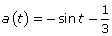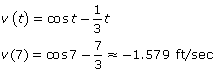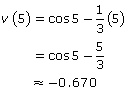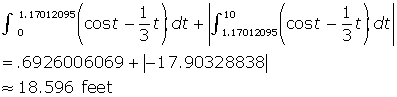# Problem 15: A Grizzly Motion Problem

2011-2012 The motion of a grizzly bear stalking its prey, walking left and right of a fixed point in feet per second, can be modeled by the motion of a particle moving left and right along the x-axis, according to the following acceleration equation: Assume that the origin corresponds to the fixed point, and that … Continue reading "Problem 15: A Grizzly Motion Problem"2011-2012

The motion of a grizzly bear stalking its prey, walking left and right of a fixed point in feet per second, can be modeled by the motion of a particle moving left and right along the x-axis, according to the following acceleration equation:Assume that the origin corresponds to the fixed point, and that a positive value for position means that the bear is located to the right of the fixed point as we watch said bear from a safe location.

If the bear’s velocity is 1 ft/sec when t = 0, answer the following questions:

(a) Identify the velocity equation that represents the bear’s motion.

(b) Determine how fast the bear was traveling at t = 7 seconds.

(c) In what direction is the bear traveling at t = 5 seconds?

(d) How far does the bear walk during the first 10 seconds?

Note: You can (and should) use a graphing calculator for part (d).

### Solution:

(a) You are given the acceleration equation. Recall that velocity is the antiderivative of acceleration, so integrate a(t) and use the fact that v(0) = 1  to identify the velocity equation.(b) Evaluate v(7).Speed is the absolute value of velocity, so the bear is traveling at a speed of 1.579 ft/sec when t = 7.

(c) Evaluate v(5).Because v(5) is negative, the bear is traveling left at t = 5.

(d) You must split the interval [0,10] into segments based on the t-intercepts of v(t). Those values of t are the times at which the bear changes direction; you must measure how far the bear traveled forward and backward separately.

The velocity equation has only one t-intercept on [0,10]: t = 1.17012095. To calculate the total distance traveled, compute the area between v(t) and the t-axis on the intervals [0,1.17012095] and [1.17012095,10] independently. When the bear is traveling left, this integral is a negative value, but you are asked to find the total distance traveled, not the final position of the bear. Therefore, both integrals must be positive values, so take the absolute value of the second definite integral.The video may take a few seconds to load.Having trouble Viewing Video content? Some browsers do not support this version – Try a different browser.